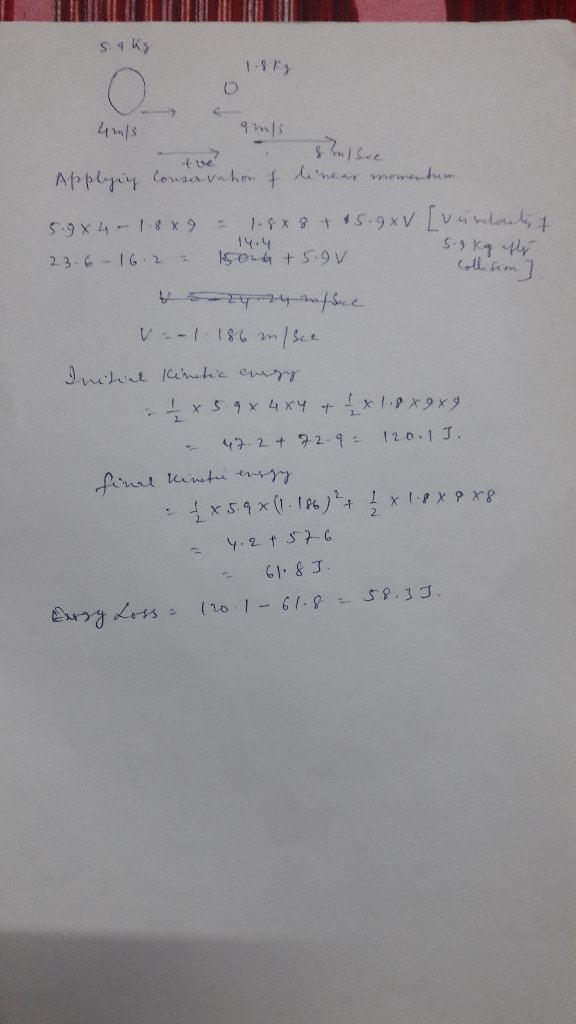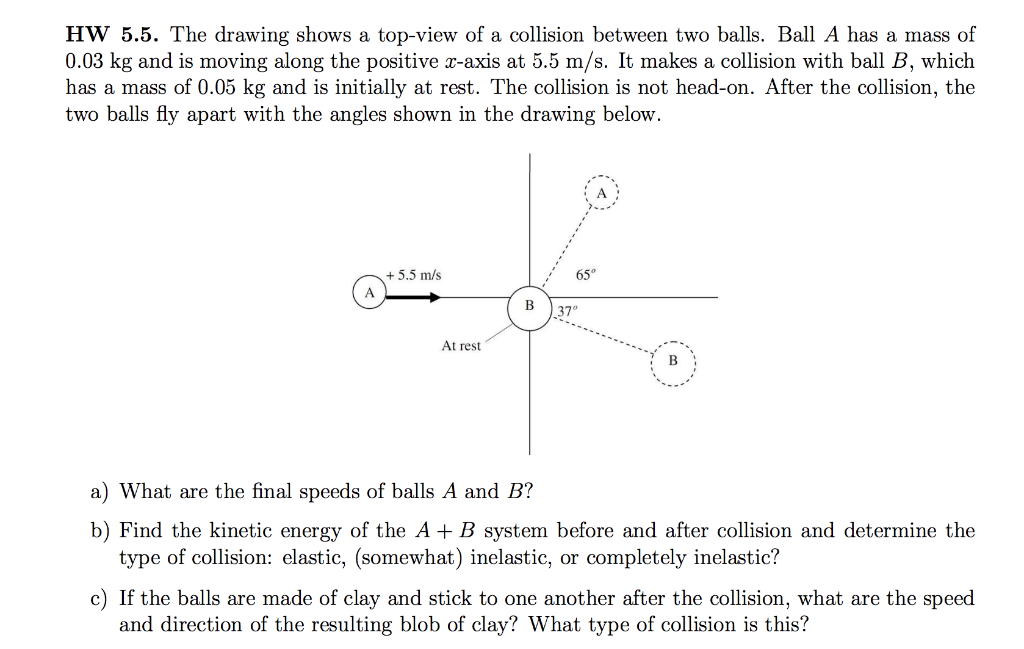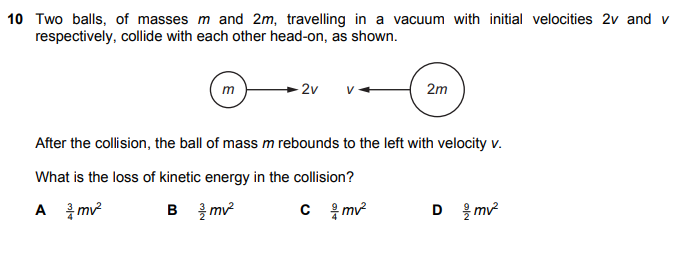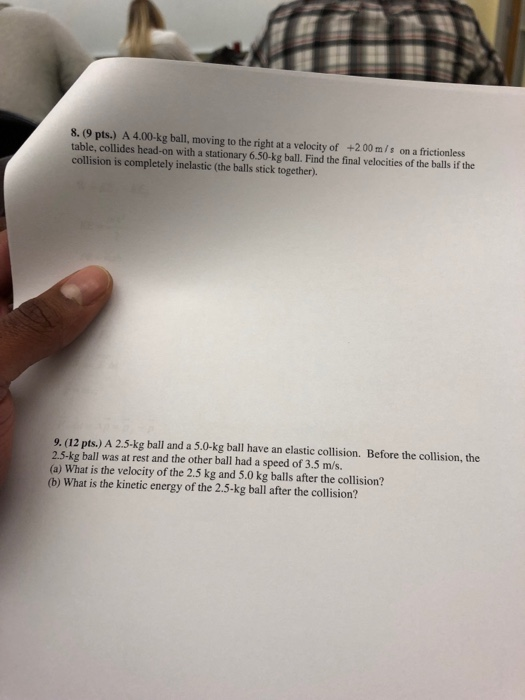Question

4. Two balls with masses of 1.80 kg and 5.90 kg travel toward each other at speeds of 9.0 m/s and 4.00 m/s, respectively. If the balls have a head-on inelastic collision and the 1.80-kilogram ball recoils with a speed of 8.00 m/s, how much kinetic energy is lost in the collision?#### Earn Coins

Coins can be redeemed for fabulous gifts.

Similar Homework Help Questions
• ### Two balls with masses of of 2.1 kg and 6.5 kg travel toward each other at...

Two balls with masses of of 2.1 kg and 6.5 kg travel toward each other at speeds of 10 m/s and 4.0 m/s, respectively. If the balls have a head-on inelastic collision and the 2.1-kilogram ball recoils with a speed of 8.00 m/s, how much kinetic energy is lost in the collision?

• ### 4. Two balls with masses of 2.30 kg and 6.30 kg travel toward each other at...4. Two balls with masses of 2.30 kg and 6.30 kg travel toward each other at speeds of 10.0 m/s and 3.80 m/s, respectively. If the balls have a head-on inelastic collision and the 2.30-kilogram ball recoils with a speed of 7.60 m/s, how much kinetic energy is lost in the collision?

• ### Two balls with masses of of 2.5 kg and 6.2 kg travel toward each other at...

Two balls with masses of of 2.5 kg and 6.2 kg travel toward each other at speeds of 9 m/s and 3.5 m/s, respectively. If the balls have a head-on inelastic collision and the 2.5-kilogram ball recoils with a speed of 7.00 m/s, how much kinetic energy is lost in the collision?

• ### Two balls with masses of of 2.1 kg and 5.9 kg travel toward each other at...

Two balls with masses of of 2.1 kg and 5.9 kg travel toward each other at speeds of 13 m/s and 4.1 m/s, respectively. If the balls have a head-on inelastic collision and the 2.1-kilogram ball recoils with a speed of 8.20 m/s, how much kinetic energy is lost in the collision?

• ### Two balls with masses of 1.50 kg and 6.30 kg travel toward each other at speeds...

Two balls with masses of 1.50 kg and 6.30 kg travel toward each other at speeds of 13.0 m/s and 4.30 m/s, respectively. If the balls have a head-on inelastic collision and the 1.50-kilogram ball recoils with a speed of 8.60 m/s, how much kinetic energy is lost in the collision?

• ### Two masses (marbles, clay, or kittens, whichever) 18 kg and 29 kg collide while traveling toward...

Two masses (marbles, clay, or kittens, whichever) 18 kg and 29 kg collide while traveling toward each other at 6 m/s each. If collision were elastic, the total kinetic energy before and after the collision would be the same. Compare the total kinetic energy before and after the collision if it were completely inelastic instead. How much is lost?

• ### HW 5.5. The drawing shows a top-view of a collision between two balls. Ball A has...HW 5.5. The drawing shows a top-view of a collision between two balls. Ball A has a mass of 0.03 kg and is moving along the positive c-axis at 5.5 m/s. It makes a collision with ball B, which has a mass of 0.05 kg and is initially at rest. The collision is not head-on. After the collision, the two balls fly apart with the angles shown in the drawing below. + 5.5 m/s At rest a) What are the...

• ### Two balls, of masses m and 2m, travelling in a vacuum with initial velocities 2v and...Two balls, of masses m and 2m, travelling in a vacuum with initial velocities 2v and v respectively, collide with each other head-on, as shown. m 2v v 2m After the collision, the ball of mass m rebounds to the left with velocity v. What is the loss of kinetic energy in the collision? A 3/4 mv^2 B 3/2 mv^2 C 9/4 mv^2 D 9/2mv^2 10 Two balls, of masses m and 2m, travelling in a vacuum with initial velocities...

• ### Q1 A small object with a momentum of magnitude 2.71 kg m/s approaches head-on a large...

Q1 A small object with a momentum of magnitude 2.71 kg m/s approaches head-on a large object at rest. The small object bounces straight back with a momentum of magnitude 6.19 kg m/s. What is the magnitude of the small object's momentum change in kg m/s? Q2 A force acts on a 4.708 kg mass as follows: the force starts at zero and rises to 60.334 N linearly in 2.24 seconds, it remains at 60.334 N for another 5.756 seconds,...

• ### 8. (9 pts.) A 4.00-kg ball, moving to the right at a velocity of +2.00 mis...8. (9 pts.) A 4.00-kg ball, moving to the right at a velocity of +2.00 mis on a frictionless table, collides head-on with a stationary 6.50-kg ball. Find the final velocities of the balls if the collision is completely inelastic (the balls stick together). 9. (12 pts.) A 2.5-kg ball and a 5.0-kg ball have an elastic collision. Before the collision, the 2.5-kg ball was at rest and the other ball had a speed of 3.5 m/s. (a) What is...